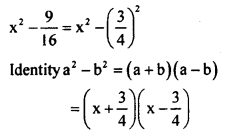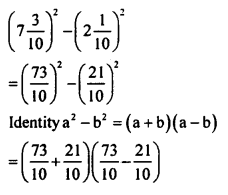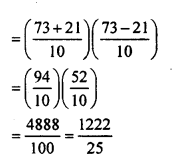# KSEEB Solutions for Class 8 Maths Chapter 4 Factorisation Ex 4.1

In this chapter, we provide KSEEB SSLC Class 8 Maths Chapter 4 Factorisation Ex 4.1 for English medium students, Which will very helpful for every student in their exams. Students can download the latest KSEEB SSLC Class 8 Maths Chapter 4 Factorisation Ex 4.1 pdf, free KSEEB SSLC Class 8 Maths Chapter 4 Factorisation Ex 4.1 pdf download. Now you will get step by step solution to each question.

## Karnataka Board Class 8 Maths Chapter 4 Factorisation Ex 4.1

1. Resolve into factors.

Question i.
x² + xy
x² + xy = x(x + y)

Question ii.
3x² – 6x
3x² – 6x = 3x (x – 2)

Question iii.
(1.6)a² – (0.8)a
(1.6)² – (0.8)a
= (0.8 x 2a²) – (0.8)a
= 0. 8a(2a- 1)

Question iv.
5 – 10m – 20n
5 – 10m -20n = 5(1 – 2m – 4n)

2. Froctorise:

Question i.
a² + ax + ab + bx
a² + ax + ab + bx a(a + x) + b(a + x)
(a + x)(a + b)

Question ii.
3ac + 7bc – 3ad – 7bd
3ac + 7bc – 3ad – 7bd
c(3a + 7b – d(3a + 7b) (3a + 7b) (c-d)

Question iii.
3xy – 6zy – 3xt + 6zt
3y (x – 2z) – 3t(x – 2z)
(x – 2z) (3y – 3t)

Question iv.
y3 + 3y² + 2y – 6 – xy + 3x
y² (y – 3) + 2(y – 3) – x(y – 3)
(y- 3) (y² + 2 – x)

3. Factorise:

Question i.
4a² – 25
4a² – 25
= (2a)² – 52
[a² – b²=(a+b)(a-b)]
= (2a + 5)(2a – 5)

Question ii.
x2−916
Ans.Question iii.
x4 – y4
x4 – y4
= (x²)² – (y²)²
Identity a² – b² = (a + b)(a – b)
= (x² + y²)(x² – y²)
= (x²+ y²)(x + y)(x – y)

Question iv.
(7310)2−(2110)2
Ans.Question v.
(0.7)² – (0.3)²
(0.7)² – (0.3)²
Identity a² – b² = (a + b)(a – b)
= (0.7 + 0.3)(0.7 – 0.3)
= (1.0)(0.4)
= 0.4

Question vi.
(5a-2b)²-(2a-b)²
(5a-2b)² – (2a-b)²
Identity a² – b² = (a + b)(a – b)
= [5a – 2b + 2a – b]
= [5a – 2b – (2a – b)]
= (7a – 3b)[5a – 2b – 2a + b]
= (7a – 3b)(3a – b)

All Chapter KSEEB Solutions For Class 8 maths

—————————————————————————–

All Subject KSEEB Solutions For Class 9

*************************************************

I think you got complete solutions for this chapter. If You have any queries regarding this chapter, please comment on the below section our subject teacher will answer you. We tried our best to give complete solutions so you got good marks in your exam.

If these solutions have helped you, you can also share kseebsolutionsfor.com to your friends.

Best of Luck!!